# Nowhere-zero flows

Open problems about Nowhere-zero flows (not to be confused with Network flows).

## 5-flow conjecture ★★★★

Author(s): Tutte

Conjecture   Every bridgeless graph has a nowhere-zero 5-flow.

Keywords: cubic; nowhere-zero flow

## 4-flow conjecture ★★★

Author(s): Tutte

Conjecture   Every bridgeless graph with no Petersen minor has a nowhere-zero 4-flow.

Keywords: minor; nowhere-zero flow; Petersen graph

## 3-flow conjecture ★★★

Author(s): Tutte

Conjecture   Every 4-edge-connected graph has a nowhere-zero 3-flow.

Keywords: nowhere-zero flow

## Jaeger's modular orientation conjecture ★★★

Author(s): Jaeger

Conjecture   Every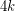-edge-connected graph can be oriented so that(mod) for every vertex.

Keywords: nowhere-zero flow; orientation

## Bouchet's 6-flow conjecture ★★★

Author(s): Bouchet

Conjecture   Every bidirected graph with a nowhere-zero-flow for some, has a nowhere-zero-flow.

Keywords: bidirected graph; nowhere-zero flow

## The three 4-flows conjecture ★★

Author(s): DeVos

Conjecture   For every graphwith no bridge, there exist three disjoint sets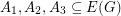withso that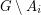has a nowhere-zero 4-flow for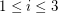.

Keywords: nowhere-zero flow

## A homomorphism problem for flows ★★

Author(s): DeVos

Conjecture   Letbe abelian groups and let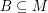andsatisfyand. If there is a homomorphism from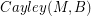to, then every graph with a B-flow has a B'-flow.

Keywords: homomorphism; nowhere-zero flow; tension

## Real roots of the flow polynomial ★★

Author(s): Welsh

Conjecture   All real roots of nonzero flow polynomials are at most 4.

Keywords: flow polynomial; nowhere-zero flow

## Unit vector flows ★★

Author(s): Jain

Conjecture   For every graphwithout a bridge, there is a flow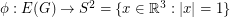.

Conjecture   There exists a map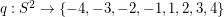so that antipodal points ofreceive opposite values, and so that any three points which are equidistant on a great circle have values which sum to zero.

Keywords: nowhere-zero flow

## Antichains in the cycle continuous order ★★

Author(s): DeVos

If,are graphs, a function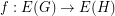is called cycle-continuous if the pre-image of every element of the (binary) cycle space ofis a member of the cycle space of.

Problem   Does there exist an infinite set of graphsso that there is no cycle continuous mapping betweenandwhenever?

Keywords: antichain; cycle; poset

## Circular flow number of regular class 1 graphs ★★

Author(s): Steffen

A nowhere-zero-flowonis an orientationoftogether with a functionfrom the edge set ofinto the real numbers such that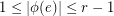, for all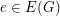, and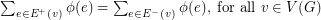. The circular flow number ofis inf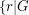has a nowhere-zero-flow, and it is denoted by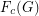.

A graph with maximum vertex degreeis a class 1 graph if its edge chromatic number is.

Conjecture   Letbe an integer anda-regular graph. Ifis a class 1 graph, then.